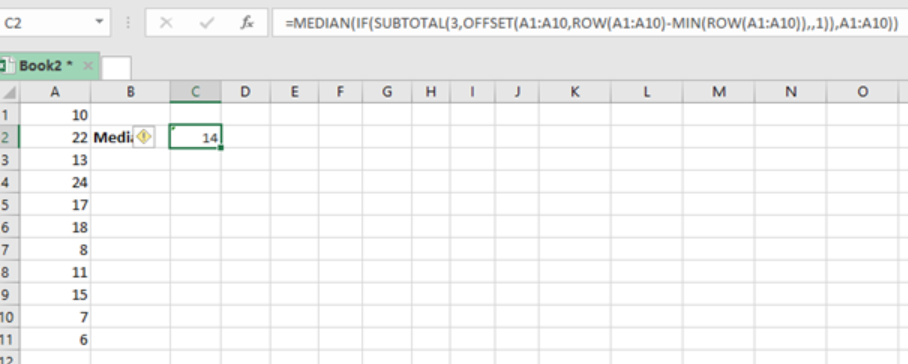Get instant live expert help with Excel or Google Sheets“My Excelchat expert helped me in less than 20 minutes, saving me what would have been 5 hours of work!”

#### Post your problem and you’ll get expert help in seconds.

Your message must be at least 40 characters
Our professional experts are available now. Your privacy is guaranteed.

# Here is How You Can Calculate the Median in Excel Using the SUBTOTAL Function

It is actually easy to calculate the median of a range of values using the MEDIAN function. But then it is not such easy when dealing with a range of values and you want to find the median that meets multiple conditions. To know how to go about this, here is a guide that can help understand how to calculate the median using the SUBTOTAL function.Figure 1: How to find median using SUBTOTAL function

## General syntax of the formula

`{=MEDIAN(IF(SUBTOTAL(3,OFFSET(A1:A10,ROW(A1:A10)-MIN(ROW(A1:A10)),,1)),A1:A10))}`

Where;

• A1:A10- refers to the range of values for which we want to find the median for.

## How the formula works

• For the above formula to work, we need to first arrange the data we have either an ascending or descending order. This is done by the MIN function in the formula.
• The IF function will then help sort our data based on the supplied criteria.
• The subtotal function will be responsible for finding the subtotals based on the criteria that we have used.
• We then use the MEDIAN function to get the median of the values, based on subtotals in a given range of values.
• It is important to note that this is an array formula, and thus should be entered in an array form. i.e. Control + Shift + Enter.

## Instant Connection to an Expert through our Excelchat Service

Most of the time, the problem you will need to solve will be more complex than a simple application of a formula or function. If you want to save hours of research and frustration, try our live Excelchat service! Our Excel Experts are available 24/7 to answer any Excel question you may have. We guarantee a connection within 30 seconds and a customized solution within 20 minutes.

Solution examplesI am having issues with max If function array formula using Is number / Find to find data
Solved by D. C. in 22 minsI need an IF statement embedded in a MIN statement to avoid the #DIV/0 return. =(MIN(1,N26/I26))
Solved by K. H. in 20 minsI need help with IF Formulas. Example. IF A2(4) is less than B2(5), I need cell C2 to read A2's number. In the same formula I need to have the option that if B2 is less than A2, that C2 needs to read B2 number.
Solved by V. J. in 17 minsI have a list of cost prices in one column and i would like to know what formula to use to add a percentage value. E.g if my cost price is just Â£1 i would like to create the sell price to be perhaps Â£3, but if my cost price is Â£1000, i would like the sell price to perhaps be Â£1,100 (not Â£3,000).
Solved by M. Y. in 60 minshello i need help creating a formula, if a value does not exsits put 0, if a value exist do nothing
Solved by M. F. in 9 mins